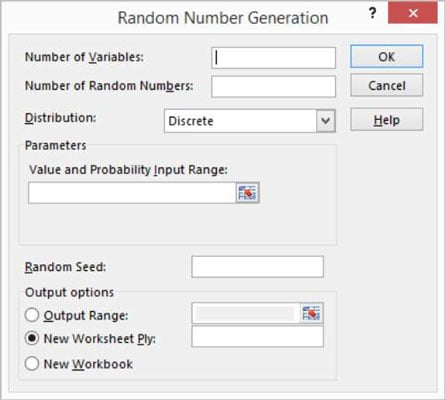##### Excel Data Analysis For DummiesThe Data Analysis command in Excel also includes a Random Number Generation tool. The Random Number Generation tool is considerably more flexible than the function, which is the other tool that you have available within Excel to produce random numbers.

The Random Number Generation tool isn’t really a tool for descriptive statistics. You would probably typically use the tool to help you randomly sample values from a population, but it’s described here because it works like the other descriptive statistics tools.

To produce random numbers, take the following steps:

1. To generate random numbers, first click the Data tab’s Data Analysis command button.

Excel displays the Data Analysis dialog box.

2. In the Data Analysis dialog box, select the Random Number Generation entry from the list and then click OK.

Excel displays the Random Number Generation dialog box.3. Describe how many columns and rows of values that you want.

Use the Number of Variables text box to specify how many columns of values you want in your output range. Similarly, use the Number of Random Numbers text box to specify how many rows of values you want in the output range.

You don't absolutely need to enter values into these two text boxes, by the way. You can also leave them blank. In this case, Excel fills all the columns and all the rows in the output range.

4. Select the distribution method.

Select one of the distribution methods from the Distribution drop-down list. The Distribution drop-down list provides several distribution methods: Uniform, Normal, Bernoulli, Binomial, Poisson, Patterned, and Discrete. Typically, if you want a pattern of distribution other than Uniform, you'll know which one of these distribution methods is appropriate.

5. (Optional) Provide any parameters needed for the distribution method.

If you select a distribution method that requires parameters, or input values, use the Parameters text box (Value and Probability Input Range) to identify the worksheet range that holds the parameters needed for the distribution method.

6. (Optional) Select a starting point for the random number generation.

You have the option of entering a value that Excel will use to start its generation of random numbers. The benefit of using a Random Seed value, as Excel calls it, is that you can later produce the same set of random numbers by planting the same “seed.”

7. Identify the output range.

Use the Output Options radio buttons to select the location that you want for random numbers.

8. After you describe how you want Excel to generate random numbers and where those numbers should be placed, click OK.

Excel generates the random numbers.